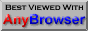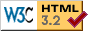# R. T. RUSSELL

## Polynomial Fitting

This program fits an order-five polynomial to a set of 20000 experimental data points. It demonstrates the power of BBC BASIC's built-in array and matrix operations to perform high-speed calculations. It could be adapted to carry out other least-squares fitting tasks.

REM POLYFIT - fit a polynomial to a set of experimental data
REM demonstrating the use of BBC BASIC's matrix operations.
REM In this version the maximum order of polynomial is five.
REM Richard T. Russell, richard@rtrussell.co.uk, 12-Apr-2011

HIMEM = PAGE + 5000000
INSTALL @lib\$+"ARRAYLIB"
INSTALL @lib\$+"FNUSING"
INSTALL @lib\$+"WINLIB5"
*FLOAT 64

file\$ = @dir\$+"polyfit.csv"
max% = 20000

REM Initialise screen:

SYS "SetWindowText", @hwnd%, "Least squares polynomial fitting"
VDU 23,22,720;480;8,16,16,128
VDU 5
ORIGIN 140,168
COLOUR 2,0,100,0
PROCaxes

REM Create controls:

button1% = FN_button("Load && plot file", 190, 12, 120, 20, FN_setproc(PROCload), 0)
button2% = FN_button("Fit polynomial", 390, 12, 120, 20, FN_setproc(PROCfit), 0)
SYS "EnableWindow", button2%, 0

REM Declare arrays:

DIM vector(5), matrix(5,5)
DIM x(max%), x2(max%), x3(max%), x4(max%), x5(max%)
DIM x6(max%), x7(max%), x8(max%), x9(max%), x10(max%)
DIM y(max%), xy(max%), x2y(max%), x3y(max%), x4y(max%), x5y(max%)

REM Idle loop:

REPEAT
WAIT
1
UNTIL FALSE
END

REM Draw axes and labels:

DEF PROCaxes
CLS
GCOL
0
FOR x = 0.0 TO 1.0 STEP 0.1
MOVE x*1200, 0 : PLOT 21, x*1200, 700
MOVE x*1200-24, -24 : PRINT FNusing("#.#", x);
NEXT
FOR
y = 0 TO 6
MOVE 0, 700*y/6 : PLOT 21, 1198, 700*y/6
MOVE -80, 700*y/6+16 : PRINT FNusing("#.#", y);
NEXT
RECTANGLE
0, 0, 1198, 700
ENDPROC

file% = OPENIN(file\$)
IF file% = 0 ERROR 0, "Could not open file " + file\$

PROCaxes
SYS "EnableWindow", button2%, 0
index% = 0
x() = 0.0
y() = 0.0
GCOL 4
WHILE NOT EOF#file% AND index% <= max%
record\$ = GET\$#file%
comma% = INSTR(record\$, ",")
IF comma% THEN

x(index%) = VAL(record\$)
y(index%) = VAL(MID\$(record\$,comma%+1))
LINE x(index%)*1200, y(index%)*700/6, x(index%)*1200, y(index%)*700/6
index% += 1
ENDIF
ENDWHILE
CLOSE
#file%
npts% = index%
SYS "EnableWindow", button2%, 1
ENDPROC

REM Fit and plot polynomial:

DEF PROCfit
TIME = 0

sum_x = SUM(x())
x2()  = x() * x()   : sum_x2  = SUM(x2())
x3()  = x() * x2()  : sum_x3  = SUM(x3())
x4()  = x2() * x2() : sum_x4  = SUM(x4())
x5()  = x2() * x3() : sum_x5  = SUM(x5())
x6()  = x3() * x3() : sum_x6  = SUM(x6())
x7()  = x3() * x4() : sum_x7  = SUM(x7())
x8()  = x4() * x4() : sum_x8  = SUM(x8())
x9()  = x4() * x5() : sum_x9  = SUM(x9())
x10() = x5() * x5() : sum_x10 = SUM(x10())

sum_y = SUM(y())
xy()  = x() * y()   : sum_xy  = SUM(xy())
x2y() = x2() * y()  : sum_x2y = SUM(x2y())
x3y() = x3() * y()  : sum_x3y = SUM(x3y())
x4y() = x4() * y()  : sum_x4y = SUM(x4y())
x5y() = x5() * y()  : sum_x5y = SUM(x5y())

matrix() = \

\ npts%,  sum_x,   sum_x2,  sum_x3,  sum_x4,  sum_x5, \

\ sum_x,  sum_x2,  sum_x3,  sum_x4,  sum_x5,  sum_x6, \

\ sum_x2, sum_x3,  sum_x4,  sum_x5,  sum_x6,  sum_x7, \

\ sum_x3, sum_x4,  sum_x5,  sum_x6,  sum_x7,  sum_x8, \

\ sum_x4, sum_x5,  sum_x6,  sum_x7,  sum_x8,  sum_x9, \

\ sum_x5, sum_x6,  sum_x7,  sum_x8,  sum_x9,  sum_x10

vector() = \

\ sum_y,  sum_xy,  sum_x2y, sum_x3y, sum_x4y, sum_x5y

PROC_invert(matrix())
vector() = matrix().vector()

took = TIME / 100

GCOL 1
MOVE 80, -72
PRINT "y = " FNusing("##.###", vector(0)) FNusing("+##.###", vector(1)) " x" \

\    FNusing("+##.###", vector(2)) " x^2" FNusing("+##.###", vector(3)) " x^3" \

\    FNusing("+##.###", vector(4)) " x^4" FNusing("+##.###", vector(5)) " x^5"

GCOL 2
MOVE 80, -120
PRINT "Fitting order-five polynomial to " ;npts% " points took " ;
PRINT FNusing("#.##", took) " seconds"

GCOL 9
FOR x = 0.0 TO 1.0 STEP 0.001
y = 0
FOR power% = 0 TO 5
y += vector(power%) * x^power%
NEXT
PLOT
x*1200, y*700/6
NEXT x

ENDPROC